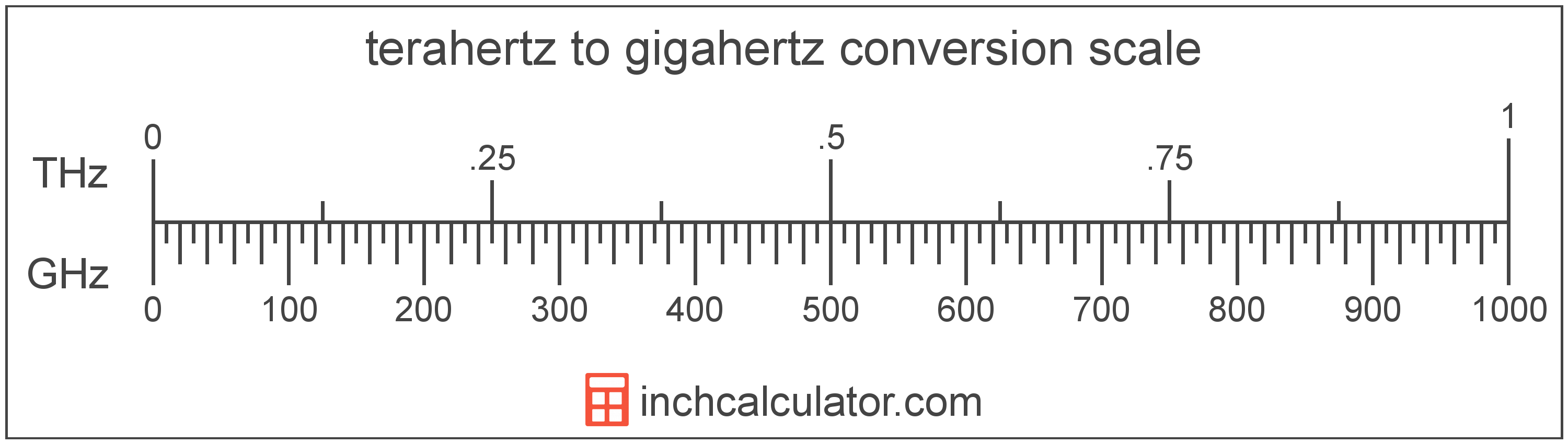# Terahertz to Gigahertz Converter

Enter the frequency in terahertz below to get the value converted to gigahertz.

Results in Gigahertz:1 THz = 1,000 GHz

Do you want to convert gigahertz to terahertz?

## How to Convert Terahertz to Gigahertz

To convert a measurement in terahertz to a measurement in gigahertz, multiply the frequency by the following conversion ratio: 1,000 gigahertz/terahertz.

Since one terahertz is equal to 1,000 gigahertz, you can use this simple formula to convert:

gigahertz = terahertz × 1,000

The frequency in gigahertz is equal to the frequency in terahertz multiplied by 1,000.

For example, here's how to convert 5 terahertz to gigahertz using the formula above.
gigahertz = (5 THz × 1,000) = 5,000 GHz### How Many Gigahertz Are in a Terahertz?

There are 1,000 gigahertz in a terahertz, which is why we use this value in the formula above.

1 THz = 1,000 GHz

## What Is a Terahertz?

Terahertz is a measure of frequency equal to one trillion cycles per second.

The terahertz is a multiple of the hertz, which is the SI derived unit for frequency. In the metric system, "tera" is the prefix for 1012. Terahertz can be abbreviated as THz; for example, 1 terahertz can be written as 1 THz.

## What Is a Gigahertz?

Gigahertz is a measure of frequency equal to one billion cycles per second.

The gigahertz is a multiple of the hertz, which is the SI derived unit for frequency. In the metric system, "giga" is the prefix for billions, or 109. Gigahertz can be abbreviated as GHz; for example, 1 gigahertz can be written as 1 GHz.

## Terahertz to Gigahertz Conversion Table

Table showing various terahertz measurements converted to gigahertz.
Terahertz Gigahertz
0.001 THz 1 GHz
0.002 THz 2 GHz
0.003 THz 3 GHz
0.004 THz 4 GHz
0.005 THz 5 GHz
0.006 THz 6 GHz
0.007 THz 7 GHz
0.008 THz 8 GHz
0.009 THz 9 GHz
0.01 THz 10 GHz
0.02 THz 20 GHz
0.03 THz 30 GHz
0.04 THz 40 GHz
0.05 THz 50 GHz
0.06 THz 60 GHz
0.07 THz 70 GHz
0.08 THz 80 GHz
0.09 THz 90 GHz
0.1 THz 100 GHz
0.2 THz 200 GHz
0.3 THz 300 GHz
0.4 THz 400 GHz
0.5 THz 500 GHz
0.6 THz 600 GHz
0.7 THz 700 GHz
0.8 THz 800 GHz
0.9 THz 900 GHz
1 THz 1,000 GHz